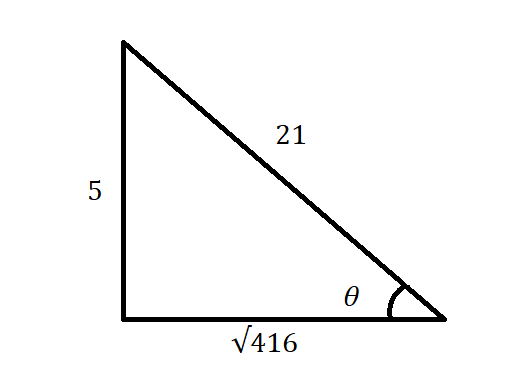bobbie71G

2021-03-06

Sketch a right triangle corresponding to the trigonometric function of the acute angle theta. Use the Pythagorean Theorem to determine the third side and then find the other five trigonometric functions of theta. $\mathrm{cos}\theta =\frac{21}{5}$Anonym

Note that, $\mathrm{csc}\theta =$ $\frac{\text{Hypotenuse}}{\text{Opposide side}}$
So $\text{Hypotenuse}=21$ and $\text{Opposite side}=5$.
Use Pythagorean theorem to find adjacent side as follows.
Adjacent side $=\sqrt{{21}^{2}-{5}^{2}}$
$=\sqrt{441-25}$
$=\sqrt{416}$
Sketch a right triangle for given trigonometric function.Find the other five trigonometric function as follows.
$\mathrm{sin}\theta =$ $\frac{\text{Opposide side}}{\text{Hypotenuse}}$ $=\frac{5}{21}$
$\mathrm{cos}\theta =$ $\frac{\text{Adjacent side}}{\text{Hypotenuse}}$ $=\frac{\sqrt{416}}{21}$
$\mathrm{tan}\theta =$ $\frac{\text{Opposide side}}{\text{Adjacent side}}$ $=\frac{5}{\sqrt{416}}$
$\mathrm{sec}\theta =$ $\frac{\text{Hypotenuse}}{\text{Adjacent side}}$ $=\frac{21}{\sqrt{416}}$
$\mathrm{cot}\theta =$ $\frac{\text{Adjacent side}}{\text{Opposide side}}$ $=\frac{\sqrt{416}}{5}$

Do you have a similar question?